﻿ 沥青混合料结构参数与蠕变特性关系分析 Analysis of Structural Parameters and Creep Characteristics of Asphalt Mixture

Open Journal of Transportation Technologies
Vol.07 No.02(2018), Article ID:24110,10 pages
10.12677/OJTT.2018.72009

Analysis of Structural Parameters and Creep Characteristics of Asphalt Mixture

Haiquan Liu1, Lun Ji1, Lei Zhang2, Jun Li1, Wendong He1,3

1School of Transportation Science and Engineering, Harbin Institute of Technology, Harbin Heilongjiang

2China Road & Bridge Co., Ltd., Beijing

3Shanxi Transportation Research Institute, Taiyuan Shanxi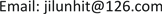Received: Feb. 28th, 2018; accepted: Mar. 14th, 2018; published: Mar. 21st, 2018ABSTRACT

The structure of asphalt mixture influences its creep characteristics. In order to study the relationship between the structure parameters and creep characteristics of asphalt mixtures, the creep tests of different gradation (5 kinds of AC and 5 kinds of SMA) asphalt mixtures were carried out, and the relationship between creep properties and structural parameters was discussed based on the Burgers creep model. The results show that the increase of coarse particles significantly improves the rheological time of AC16 and SMA16, and enhances the resistance to permanent deformation of asphalt mixtures. The correlation between void fraction Q and η1 is significant in AC16 structural parameters. In SMA16 structural parameters, coarse-grained area, void fraction, parameter Q and trend parameter Δ are significantly correlated with η1 and η2. The increase of 4.75 passing rate reversed the change of the rheological time of AC16 and SMA16.

Keywords:Structural Parameters of Asphalt Mixture, Creep, Rheological Time, Permanent Deformation Resistance

1哈尔滨工业大学交通科学与工程学院，黑龙江 哈尔滨

2中国路桥工程有限责任公司，北京

3山西省交通科学研究院，山西 太原1. 引言

2. 试验材料及沥青混合料设计

2.1. 试验选用的级配

2.2. 沥青用量Table 1. Pass rate of 5 kinds of gradation (J1 - J5)Table 2. Gradation with the best asphalt value of the amount of asphalt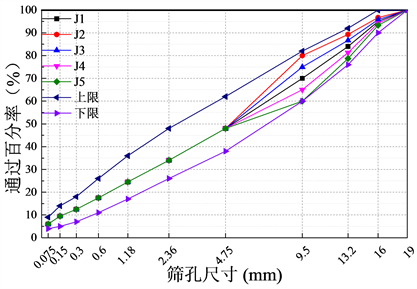Figure 1. AC16 different coarse aggregate gradation curve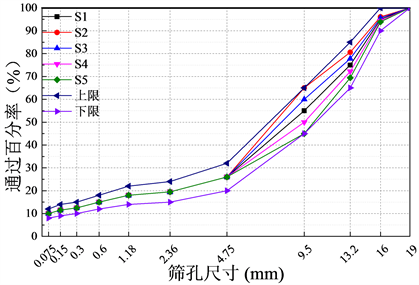Figure 2. SMA16 different coarse aggregate gradation curve

3. 蠕变试验与结构参数

3.1. 蠕变试验和分析模型

1) 蠕变试验

$\epsilon \left(t\right)={\sigma }_{0}\left[\frac{1}{{E}_{1}}+\frac{1}{{\eta }_{1}}t+\frac{1}{{E}_{2}}\left(1-{\text{e}}^{-\frac{{E}_{2}}{{\eta }_{2}}t}\right)\right]$ (1)

${E}_{1}$ ——瞬时弹性模量(MPa)；

${E}_{2}$ ——延迟弹性模量(MPa)；

${\eta }_{1},{\eta }_{2}$ ——粘壶粘性系数。

2) 分析模型

Burgers模型能很好的对蠕变曲线的迁移期和稳定期进行拟合，但是不能就破坏期进行拟合。因此，下文主要就蠕变曲线的前两个阶段进行拟合分析。蠕变曲线如图3所示。

3.2. 结构参数量化单位

1) 纵横比 $A{R}_{1}$ ：将集料颗粒最小外接矩形的长轴与短轴的比值称为纵横比 $A{R}_{1}$ ，如图4，定义式如式所示：

$A{R}_{1}=\frac{{D}_{1}}{{D}_{2}}$ (2)

${D}_{2}$ ——最小外接矩形短轴(cm)。

2) 棱角性：棱角性的定义主要分为两类，第一类是反映颗粒轮廓上棱角的圆度，第二类是反映颗粒轮廓的整体轮廓的圆度。本文采用第二类基于颗粒周长计算的棱角性指标，用颗粒实际周长与等效椭圆的周长的比值表征颗粒的棱角性  ，如图4示意，表达式如式(3)所示：

$Angularity\left(P\right)={\left(\frac{Perimeter}{Perimete{r}_{ellipse}}\right)}^{2}$ (3)

$Perimete{r}_{ellipse}$ ——等效椭圆周长(cm)。

3) 集料面积(Area)：集料面积可分为颗粒实际面积、等效椭圆面积和最小外接矩形面积Area (Box)等，本文主要针对颗粒二维实际截面进行分析。

4) 分形维数(Fractal Dimension)：分形维数则是判断两个分形是否一致的度量标准之一。本文采用IPP测量分形维数，采用变尺码法来进行分形维数的测定，如图5所示，具体如下：Figure 3. Schematic diagram of creep(a) 等效椭圆示意图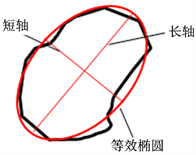(b) 最小外接矩形示意图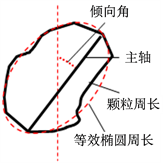(c) 棱角性示意图

Figure 4. Coarse aggregate particle size parameters of the sketch map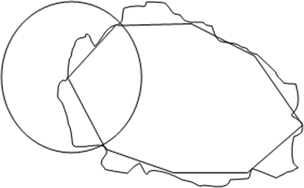Figure 5. Fractal dimension solution diagram

$L=k{\epsilon }^{1-D}$ (4)

$\mathrm{lg}L=\mathrm{lg}k+\left(1-D\right)\mathrm{lg}\epsilon$ (5)

5) 空隙轮廓分形维数

6) 空隙面积比

$Q=\frac{Are{a}_{jiliao}}{Are{a}_{kongxi}}$ (6)

$Are{a}_{kongxi}$ ——空隙横断面积(cm2)。

7) Eyad Masad  提出了集料偏角均值和颗粒趋向参数 $\Delta$ 表示集料颗偏角分布特性表达式见式(7)~(8)。

$\overline{\alpha }=\frac{\sum _{i=1}^{N}{\alpha }_{k}}{N}$ (7)

$\Delta =\frac{100}{N}\sqrt{{\left(\sum _{1}^{N}\mathrm{sin}2{\alpha }_{k}\right)}^{2}+{\left(\sum _{1}^{N}\mathrm{cos}2{\alpha }_{k}\right)}^{2}}$ (8)

${\alpha }_{k}$ ——单个颗粒主轴偏向角；

$N$ ——颗粒个数。

$\Delta$ 表示主轴分布一致性，数值在0~100之间，分布一致性越高，数值越大。

4. AC16级配沥青混合料结构参数和性能分析

4.1. AC16级配沥青混合料流变时间和蠕变特性

AC16不同级配的沥青混合料流变时间和蠕变曲线如下

4.2. AC16沥青混合料结构参数与蠕变性能相关性分析

AC16级配J1~J5沥青混合料结构参数和蠕变参数相关系数统计见表4，由表中数据可知，结构参数除空隙分形维数外其他参数与Burgers蠕变模型参数和均具有很好的相关性，与相关性次之，最差。由此可知，在AC类沥青混合料中，集料颗粒面积的增加，集料分形维数的降低，空隙率的降低能够提高粘性、和，提高其永久变能力。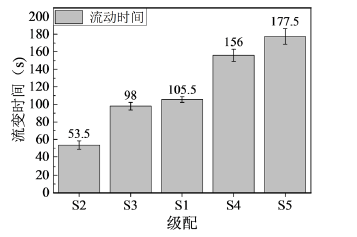J1~J5 (60℃, 0.4 MPa)

Figure 6. Different grades of asphalt mixture rheological time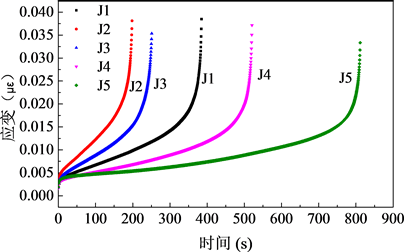Figure 7. J1~J5 asphalt mixture creep curveTable 3. AC16 asphalt mixture structure and rheological time correlation analysisTable 4. J1~J5 structural parameters and creep parameters correlation coefficient

5. SMA16级配沥青混合料结构参数和性能分析

5.1. SMA16级配沥青混合料流变时间和蠕变特性

SMA16不同粗集料级配沥青混合料流变时间和蠕变曲线统计结果如下：

SMA16沥青混合料结构参数和流变时间的相关系数计算统计见表5

5.2. SMA16沥青混合料结构参数与蠕变性能相关性分析

S1~S5沥青混合料结构参数和蠕变参数相关系数统计见表6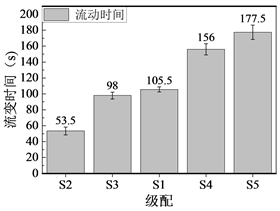Figure 8. Different grades with asphalt mixture rheological time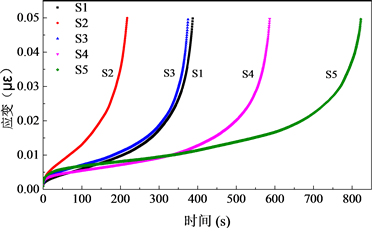Figure 9. S1~S5 asphalt mixture creep curveTable 5. SAM-16 Asphalt Mixture Structure and Rheological Correlation AnalysisTable 6. S1~S5 structural parameters and creep parameters correlation coefficient

6. 结论

1) 试验结果表明，蠕变试验试件的破坏形式为“两端细、中间粗”的鼓状破坏形式，AC16试件表面有较多的条状裂纹，SMA则表现为粗骨料之间互相错位。

2) 粗集料级配对沥青混合料流变时间具有显著影响，无论是AC类还是SMA类沥青混合料，大颗粒粗颗粒的增加都显著提高了沥青混合料的流变时间，增强了沥青混合料抗永久变形能力。

3) 在AC16中，4.75 mm通过率的降低使得流变时间降低，但对于SMA16来说，4.75 mm通过率的增加使得流动时间大大增加，与AC16呈现相反趋势。

4) AC16沥青混合料中，粗集料大颗粒的增加主要对蠕变参数 ${\eta }_{1}$${\eta }_{2}$ 产生了影响，4.75通过率的降低使得 ${\eta }_{1}$${\eta }_{2}$ 都增大，空隙率和 $Q$${\eta }_{1}$ 相关性显著。SMA16沥青混合料中，粗集料大颗粒的增加使得 ${\eta }_{1}$${\eta }_{2}$ 都增大，粗颗粒面积、空隙率、参数 $Q$ 以及趋向参数 $\Delta$${\eta }_{1}$${\eta }_{2}$ 显著相关。空隙率与 ${\eta }_{1}$ 负相关显著，参数 $Q$${\eta }_{1}$ 正相关显著。

5) 抗永久变形性能与粗集料面积参数、空隙分形维数、横向 $\Delta$ 、纵向 $\Delta$ 、空隙率以及 $Q$ 相关性良好。

Analysis of Structural Parameters and Creep Characteristics of Asphalt Mixture[J]. 交通技术, 2018, 07(02): 69-78. https://doi.org/10.12677/OJTT.2018.72009

1. 1. 谭忆秋. 沥青与沥青混合料[M]. 哈尔滨: 哈尔滨工业大学出版社, 2007: 52-54.

2. 2. 卢永贵, 张登良. 粗集料间隙率试验研究[J]. 长安大学学报(自然科学版), 2001, 21(1): 33-36.

3. 3. 吴旷怀, 张肖宁. 沥青混合料设计综述[J]. 广州大学学报(自然科学版), 2005, 4(5): 456-461.

4. 4. 徐慧宁. 体积指标对沥青混合料路用性能的影响[D]: [硕士学位论文]. 哈尔滨: 哈尔滨工业大学, 2007.

5. 5. 贾渝. Superpave混合料设计方法最新进展[J]. 中外公路, 2001, 21(6): 58-61.

6. 6. ASTM D3398-2000. Test Method for the Index of Aggregate Particle Shape and Texture. Annual Book of ASTM (American Society for Testing and Materials) Standards, 2000.

7. 7. Rao, C., Tutumluer, E. and Kim, I.T. (2002) Quantification of Coarse Aggregate Angularity Based on Image Analysis. Transportation Research Record Journal of the Transportation Research Board, 1787, 117-124. https://doi.org/10.3141/1787-13

8. 8. 陈国明. 沥青混合料中粗集料表面物理特性的研究[D]: [博士学位论文]. 哈尔滨: 哈尔滨工业大学, 2005.

9. 9. Chen, J.S., Wong, S.Y. and Lin, K.Y. (2005) Quantification of Movements of Flat and Elongated Particles in Hot Mix Asphalt Subject to Wheel Load Test. Materials and Structures, 38, 395-402. https://doi.org/10.1007/BF02479307

10. 10. Delrio-Prat, M., Vega-Zamanillo, A., Castro-Fresno, D., et al. (2011) Energy Consumption during Compaction with a Gyratory Intensive Compactor Tester. Estimation Models. Construction & Building Materials, 25, 979-986. https://doi.org/10.1016/j.conbuildmat.2010.06.083

11. 11. Buchanan, M.S. (2000) Evaluation of the Effect of Flat and Elongated Particles on the Performance of Hot Mix Asphalt Mixtures. Coarse Aggregates.

12. 12. 陈建旭, 林桂仪, 陈虹洁. 粒料对沥青混凝土性能之影响[C]. 全国公路科技创新高层论坛, 2004: 2-9.

13. 13. 谭忆秋, 宋宪辉, 纪伦, 等. 粗集料性能对沥青混合料高温性能的影响[J]. 中国公路学报, 2009(1): 29-33.

14. 14. 彭波. 沥青混合料集料几何特性与结构研究[D]: [博士学位论文]. 西安: 长安大学道路与铁道工程, 2008.

15. 15. 赵永利, 黄晓明. 矿料级配基本性能的试验研究[J]. 公路交通科技, 2004, 21(6): 1-3.

16. 16. Masad, E., Muhunthan, B., Shashidhar, N., et al. (1999) Quantifying Laboratory Compaction Effects on the Internal Structure of Asphalt Concrete. Transportation Research Record, 1, 185-197.